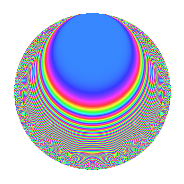# Properties

 Label 445.2.aLevel 445 Weight 2 Character orbit a Rep. character $$\chi_{445}(1,\cdot)$$ Character field $$\Q$$ Dimension 29 Newforms 7 Sturm bound 90 Trace bound 3

# Related objects

## Defining parameters

 Level: $$N$$ = $$445 = 5 \cdot 89$$ Weight: $$k$$ = $$2$$ Character orbit: $$[\chi]$$ = 445.a (trivial) Character field: $$\Q$$ Newforms: $$7$$ Sturm bound: $$90$$ Trace bound: $$3$$ Distinguishing $$T_p$$: $$2$$

## Dimensions

The following table gives the dimensions of various subspaces of $$M_{2}(\Gamma_0(445))$$.

Total New Old
Modular forms 46 29 17
Cusp forms 43 29 14
Eisenstein series 3 0 3

The following table gives the dimensions of the cuspidal new subspaces with specified eigenvalues for the Atkin-Lehner operators and the Fricke involution.

$$5$$$$89$$FrickeDim.
$$+$$$$+$$$$+$$$$6$$
$$+$$$$-$$$$-$$$$8$$
$$-$$$$+$$$$-$$$$8$$
$$-$$$$-$$$$+$$$$7$$
Plus space$$+$$$$13$$
Minus space$$-$$$$16$$

## Trace form

 $$29q$$ $$\mathstrut -\mathstrut q^{2}$$ $$\mathstrut +\mathstrut 23q^{4}$$ $$\mathstrut +\mathstrut q^{5}$$ $$\mathstrut -\mathstrut 4q^{6}$$ $$\mathstrut -\mathstrut 8q^{7}$$ $$\mathstrut -\mathstrut 9q^{8}$$ $$\mathstrut +\mathstrut 33q^{9}$$ $$\mathstrut +\mathstrut O(q^{10})$$ $$29q$$ $$\mathstrut -\mathstrut q^{2}$$ $$\mathstrut +\mathstrut 23q^{4}$$ $$\mathstrut +\mathstrut q^{5}$$ $$\mathstrut -\mathstrut 4q^{6}$$ $$\mathstrut -\mathstrut 8q^{7}$$ $$\mathstrut -\mathstrut 9q^{8}$$ $$\mathstrut +\mathstrut 33q^{9}$$ $$\mathstrut -\mathstrut q^{10}$$ $$\mathstrut -\mathstrut 4q^{11}$$ $$\mathstrut +\mathstrut 4q^{12}$$ $$\mathstrut -\mathstrut 14q^{13}$$ $$\mathstrut +\mathstrut 12q^{14}$$ $$\mathstrut -\mathstrut 4q^{15}$$ $$\mathstrut +\mathstrut 7q^{16}$$ $$\mathstrut +\mathstrut 6q^{17}$$ $$\mathstrut +\mathstrut 7q^{18}$$ $$\mathstrut +\mathstrut 7q^{20}$$ $$\mathstrut +\mathstrut 4q^{22}$$ $$\mathstrut -\mathstrut 12q^{23}$$ $$\mathstrut +\mathstrut 8q^{24}$$ $$\mathstrut +\mathstrut 29q^{25}$$ $$\mathstrut -\mathstrut 6q^{26}$$ $$\mathstrut -\mathstrut 12q^{27}$$ $$\mathstrut -\mathstrut 32q^{28}$$ $$\mathstrut -\mathstrut 2q^{29}$$ $$\mathstrut -\mathstrut 8q^{30}$$ $$\mathstrut -\mathstrut 16q^{31}$$ $$\mathstrut -\mathstrut 29q^{32}$$ $$\mathstrut +\mathstrut 28q^{33}$$ $$\mathstrut -\mathstrut 10q^{34}$$ $$\mathstrut -\mathstrut 4q^{35}$$ $$\mathstrut -\mathstrut 21q^{36}$$ $$\mathstrut -\mathstrut 26q^{37}$$ $$\mathstrut -\mathstrut 48q^{38}$$ $$\mathstrut -\mathstrut 44q^{39}$$ $$\mathstrut -\mathstrut 9q^{40}$$ $$\mathstrut +\mathstrut 2q^{41}$$ $$\mathstrut +\mathstrut 16q^{42}$$ $$\mathstrut -\mathstrut 8q^{43}$$ $$\mathstrut -\mathstrut 8q^{44}$$ $$\mathstrut +\mathstrut 5q^{45}$$ $$\mathstrut -\mathstrut 8q^{46}$$ $$\mathstrut +\mathstrut 4q^{47}$$ $$\mathstrut +\mathstrut 20q^{48}$$ $$\mathstrut +\mathstrut 13q^{49}$$ $$\mathstrut -\mathstrut q^{50}$$ $$\mathstrut +\mathstrut 24q^{51}$$ $$\mathstrut -\mathstrut 42q^{52}$$ $$\mathstrut -\mathstrut 2q^{53}$$ $$\mathstrut +\mathstrut 4q^{55}$$ $$\mathstrut -\mathstrut 4q^{56}$$ $$\mathstrut +\mathstrut 40q^{57}$$ $$\mathstrut +\mathstrut 10q^{58}$$ $$\mathstrut -\mathstrut 28q^{60}$$ $$\mathstrut -\mathstrut 14q^{61}$$ $$\mathstrut +\mathstrut 24q^{62}$$ $$\mathstrut -\mathstrut 28q^{63}$$ $$\mathstrut -\mathstrut 33q^{64}$$ $$\mathstrut +\mathstrut 2q^{65}$$ $$\mathstrut -\mathstrut 20q^{66}$$ $$\mathstrut -\mathstrut 20q^{67}$$ $$\mathstrut +\mathstrut 66q^{68}$$ $$\mathstrut +\mathstrut 36q^{69}$$ $$\mathstrut -\mathstrut 4q^{70}$$ $$\mathstrut +\mathstrut 75q^{72}$$ $$\mathstrut +\mathstrut 14q^{73}$$ $$\mathstrut -\mathstrut 14q^{74}$$ $$\mathstrut +\mathstrut 24q^{76}$$ $$\mathstrut +\mathstrut 20q^{77}$$ $$\mathstrut +\mathstrut 52q^{78}$$ $$\mathstrut -\mathstrut 36q^{79}$$ $$\mathstrut -\mathstrut q^{80}$$ $$\mathstrut +\mathstrut 45q^{81}$$ $$\mathstrut -\mathstrut 2q^{82}$$ $$\mathstrut +\mathstrut 12q^{83}$$ $$\mathstrut +\mathstrut 20q^{84}$$ $$\mathstrut -\mathstrut 14q^{85}$$ $$\mathstrut +\mathstrut 16q^{86}$$ $$\mathstrut -\mathstrut 28q^{87}$$ $$\mathstrut -\mathstrut 12q^{88}$$ $$\mathstrut +\mathstrut q^{89}$$ $$\mathstrut +\mathstrut 7q^{90}$$ $$\mathstrut +\mathstrut 20q^{91}$$ $$\mathstrut -\mathstrut 12q^{92}$$ $$\mathstrut -\mathstrut 20q^{93}$$ $$\mathstrut -\mathstrut 12q^{94}$$ $$\mathstrut -\mathstrut 12q^{95}$$ $$\mathstrut +\mathstrut 20q^{96}$$ $$\mathstrut +\mathstrut 2q^{97}$$ $$\mathstrut +\mathstrut 3q^{98}$$ $$\mathstrut -\mathstrut 16q^{99}$$ $$\mathstrut +\mathstrut O(q^{100})$$

## Decomposition of $$S_{2}^{\mathrm{new}}(\Gamma_0(445))$$ into irreducible Hecke orbits

Label Dim. $$A$$ Field CM Traces A-L signs $q$-expansion
$$a_2$$ $$a_3$$ $$a_5$$ $$a_7$$ 5 89
445.2.a.a $$2$$ $$3.553$$ $$\Q(\sqrt{5})$$ None $$-2$$ $$-2$$ $$-2$$ $$2$$ $$+$$ $$+$$ $$q-q^{2}+(-1-\beta )q^{3}-q^{4}-q^{5}+(1+\cdots)q^{6}+\cdots$$
445.2.a.b $$2$$ $$3.553$$ $$\Q(\sqrt{3})$$ None $$0$$ $$2$$ $$2$$ $$-2$$ $$-$$ $$+$$ $$q+\beta q^{2}+(1+\beta )q^{3}+q^{4}+q^{5}+(3+\beta )q^{6}+\cdots$$
445.2.a.c $$2$$ $$3.553$$ $$\Q(\sqrt{2})$$ None $$2$$ $$0$$ $$2$$ $$0$$ $$-$$ $$+$$ $$q+(1+\beta )q^{2}-\beta q^{3}+(1+2\beta )q^{4}+q^{5}+\cdots$$
445.2.a.d $$4$$ $$3.553$$ 4.4.725.1 None $$1$$ $$-2$$ $$-4$$ $$2$$ $$+$$ $$+$$ $$q+(1-\beta _{1}-\beta _{2})q^{2}+(-1+\beta _{2})q^{3}+\cdots$$
445.2.a.e $$4$$ $$3.553$$ 4.4.8069.1 None $$1$$ $$4$$ $$4$$ $$12$$ $$-$$ $$+$$ $$q+\beta _{1}q^{2}+(1-\beta _{1}+\beta _{3})q^{3}+(1+\beta _{2}+\cdots)q^{4}+\cdots$$
445.2.a.f $$7$$ $$3.553$$ $$\mathbb{Q}[x]/(x^{7} - \cdots)$$ None $$-4$$ $$-8$$ $$7$$ $$-16$$ $$-$$ $$-$$ $$q+(-1+\beta _{1})q^{2}+(-1-\beta _{5})q^{3}+(2+\cdots)q^{4}+\cdots$$
445.2.a.g $$8$$ $$3.553$$ $$\mathbb{Q}[x]/(x^{8} - \cdots)$$ None $$1$$ $$6$$ $$-8$$ $$-6$$ $$+$$ $$-$$ $$q+\beta _{1}q^{2}+(1-\beta _{3})q^{3}+(1-\beta _{1}-\beta _{3}+\cdots)q^{4}+\cdots$$

## Decomposition of $$S_{2}^{\mathrm{old}}(\Gamma_0(445))$$ into lower level spaces

$$S_{2}^{\mathrm{old}}(\Gamma_0(445)) \cong$$ $$S_{2}^{\mathrm{new}}(\Gamma_0(89))$$$$^{\oplus 2}$$